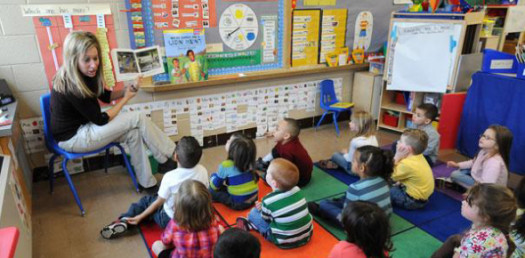# Elementary English Grammar Trivia Quiz!

10 Questions | Total Attempts: 282SettingsBelow is an Elementary English grammar trivia quiz for those of us who are having a hard time when it comes to writing or reading sentences. In this quiz, you will be expected to use either the words how many or how much. Take it up if you need the practice and take us much time as you need to choose the answer.

• 1.
We need some tea. __________________do we need?
• A.

How much

• B.

How many

• 2.
We need some eggs. __________________do we need?
• A.

How much

• B.

How many

• 3.
We need some paper. __________________do we need?
• A.

How much

• B.

How many

• 4.
We need some money. __________________do we need?
• A.

How much

• B.

How many

• 5.
__________________tea bags are there left in the box?
• A.

How much

• B.

How many

• 6.
__________________cigarettes do you smoke every day?
• A.

How much

• B.

How many

• 7.
__________________packets of cigarettes do you have?
• A.

How much

• B.

How many

• 8.
__________________work have you got to do?
• A.

How much

• B.

How many

• 9.
__________________sleep did you get last night?
• A.

How much

• B.

How many

• 10.
__________________children have you got?
• A.

How much

• B.

How many

Related TopicsBack to top